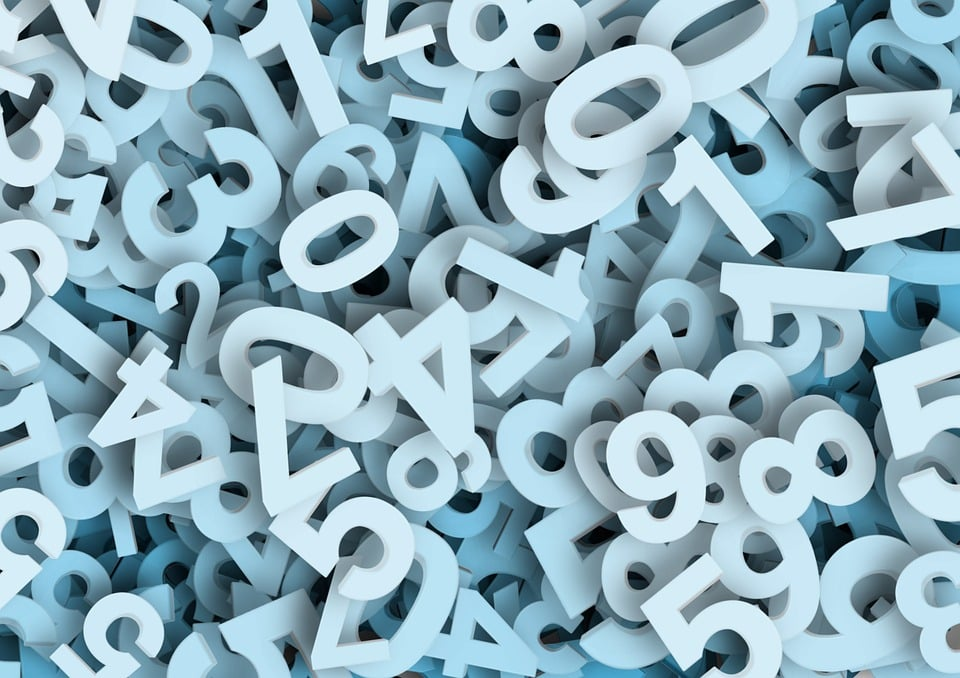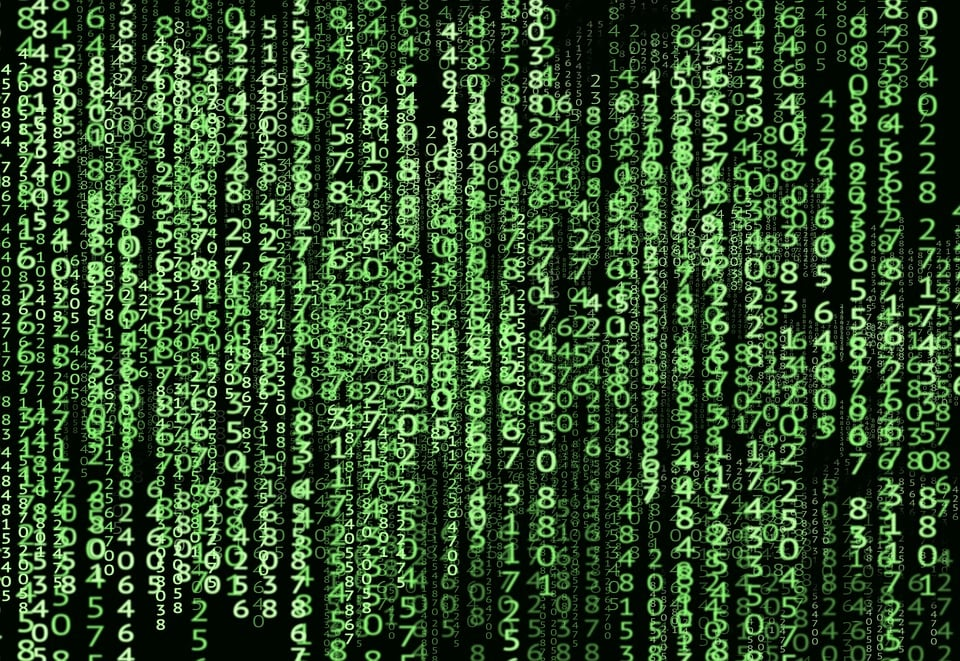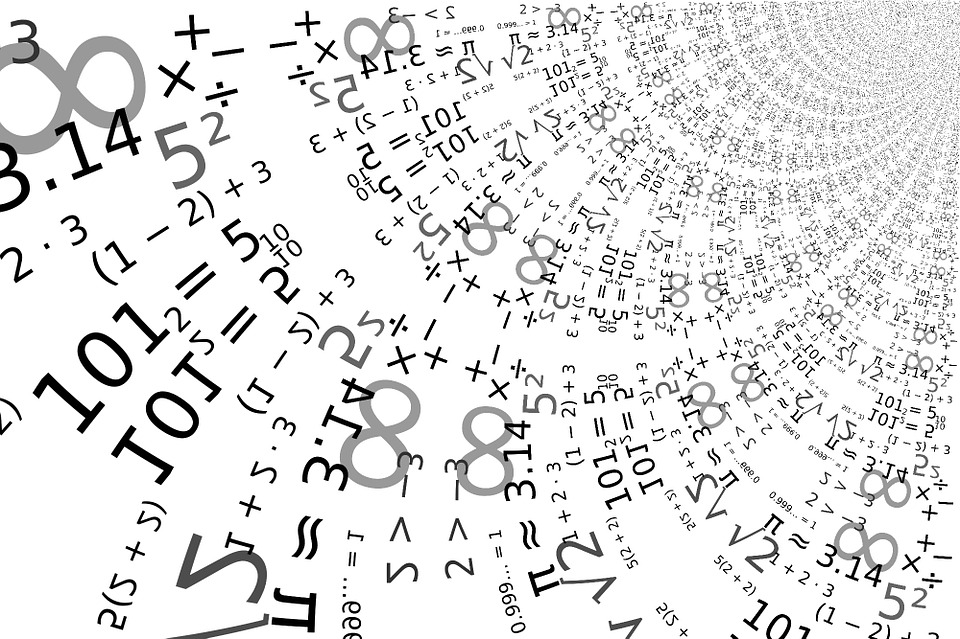### Hex:

Ascii to Hex tool uses plain text characters in ASCII  as input and converts it to hexadecimal digits. It is an online tool that is completely cost-free.Students, computer engineers, and teachers can use this converter anywhere on their mobile devices because it is a browser-based tool. This ASCII code converter is very efficient in converting ASCII to hex.

## How to use ascii to hex converter?

The ASCII to Hex converter makes it possible to convert ASCII characters into hexadecimal without clicking anywhere and waiting for long periods of page reloading as happens on other sites.

To use this converter, follow the below steps:

• Enter your ASCII value in the given input box.
• See the hexadecimal numbers in the result tab.

The ASCII decoder will instantly give you a hex number output. If you need to convert Hex back to ASCII, you can use our Hex to ASCII converter.

## How do I convert ASCII to Hex?

1. Identify the ASCII string that you want to convert to hex.
2. Use our ASCII table below to get a hexadecimal number against each character in order to save your time.
3. By repeating these steps, convert ASCII characters to hex characters.

### EXAMPLE:

Convert to hex: HEY

Let us convert the ASCII word “HEY” in capital to hexadecimal.

Step 1: Use our converter or check the ASCII table for each character in the text “HEY” in order to make sure you have received the accurate out.

By using the ASCII table below, you can find the matching characters easily.

H = 48, E = 45, Y = 59

Step 2: Use these numbers to form a hex string.

HEY = 48 45 59

So, the ASCII “HEY” is equal to the 484559 in hexadecimal.

Note: The hex value for “hey” and “HEY” are not same since each ascii character has a different value in hex. So, capital letters have different value as compare to small letters.

## ASCII Numbering System

One of the formats used to represent numbers in the form of characters is this Numbering Scheme. The ‘American Uniform Code for Interchange of Information’ is abbreviated. This is the basic code for which the characters have a specific set of notations. This method of coding is commonly preferred on computers where text files are used.The ASCII Numbering Scheme is an interlink between the system hardware and the computer screen of the user. It is considered to be a common language for computers. It is composed of the American Uniform Code for Information Interchange. You can configure and encrypt the text in the form of certain codes.

The 128 characters present in the English language are coded as numbers. Each character is assigned a number from 0 to 127. These codes are preferred in order to allow data to be transferred from one computer to another. The name indicates that these codes contain a mixture of alphabets and numbers. In general, these types of codes are preferred for data transmission.

This format is preferred by operating systems such as ‘UNIX & DOS.’ Usually, these codes are based on the roots of telegraphic codes.

The hexadecimal numbers are 0-9, then use the letters A-F. Hexadecimal is a convenient way to express binary numbers in modern computers where the byte is almost always defined as containing eight binary digits.Hexadecimal is describing the base-16 number system. That is, it describes a numbering system containing 16 sequential numbers as basic units, including 0. We use base “16” here as a decimal number to explain a number that would be “10” in hexadecimal.

This numbering scheme is equivalent to other basic systems, including binary, octal, and decimal. This is also referred to as data coding. But it’s a user-friendly language. It is very efficient in terms of storing large units of information. The conversion is also made easier with the help of the conversion table. It is known as the ‘foundation 16’ method.

## ASCII Hex table

Use the below ASCII to hex conversion table to convert ASCII to hexadecimal.

 ASCII Hexadecimal NUL 00 SOH 01 STX 02 ETX 03 EOT 04 ENQ 05 ACK 06 BEL 07 BS 08 HT 09 LF 0A VT 0B FF 0C CR 0D SO 0E SI 0F DLE 10 DC1 11 DC2 12 DC3 13 DC4 14 NAK 15 SYN 16 ETB 17 CAN 18 EM 19 SUB 1A ESC 1B FS 1C GS 1D RS 1E US 1F Space 20 ! 21 “ 22 # 23 \$ 24 % 25 & 26 ‘ 27 ( 28 ) 29 * 2A + 2B , 2C – 2D . 2E / 2F 0 30 1 31 2 32 3 33 4 34 5 35 6 36 7 37 8 38 9 39 : 3A ; 3B < 3C = 3D > 3E ? 3F @ 40 A 41 B 42 C 43 D 44 E 45 F 46 G 47 H 48 I 49 J 4A K 4B L 4C M 4D N 4E O 4F P 50 Q 51 R 52 S 53 T 54 U 55 V 56 W 57 X 58 Y 59 Z 5A [ 5B \ 5C ] 5D ^ 5E _ 5F ` 60 a 61 b 62 c 63 d 64 e 65 f 66 g 67 h 68 i 69 j 6A k 6B l 6C m 6D n 6E o 6F p 70 q 71 r 72 s 73 t 74 u 75 v 76 w 77 x 78 y 79 z 7A { 7B | 7C } 7D ~ 7E DEL 7F

## Why to convert ascii in hex?

In a computer, all data is stored and calculated using a binary representation, because the computer uses high and low levels of 1 and 0 respectively. For example, 52 letters such as a, b, c, and d, including capitals, and numbers such as 0, 1, etc., as well as some commonly used symbols (such as *, #, @, etc.) are also represented on the computer using binary numbers.

Of course, everyone can agree on their own set that this is called coding, and if everyone wants to communicate with each other without confusion, then everyone must apply the same coding rules, so that the relevant US standardisation organisations have introduced ASCII codes. Uniformly specifies which binary symbols represent the common symbols mentioned above.

Hexadecimal is a representation of data on a computer. It’s different from the representation in our daily lives. It consists of 0-9, A-F, and the letter is case-insensitive. Decimal correspondences are: 0-9 corresponds to 0-9; A-F corresponds to 10-15; N-ar numbers may be expressed as 0-(N-1) numbers and more than 9 A-F letters.

If you are a programmer, you are probably used to seeing hexadecimal notation pop up in tonnes of places. For example, hexadecimal is used to denote colours in the hex scheme. Values are also often in hexadecimal for memory readouts. Braille is coded in hexadecimal.

There are a few obvious reasons why hexadecimal is preferable to the standard binary that computers store at a low level.

Hexadecimal uses digits that more closely resemble our usual base-10 counting system, making it easier to decide at a glance how big a number like e7 is compared to 11100111.

### 2. Higher information density:

With 2 hex digits, any number from 0 to 255 can be expressed. We need 8 digits to do the same in binary. As we get bigger and bigger numbers, we’re starting to need more and more digits, and it’s getting harder to deal with.

### 3. Human friendly:

The main reason why we use hexadecimal numbers is because it provides a more human-friendly representation and is much easier to express binary number representations in hex than it is in any other base number system.
For example, a single byte can have values ranging from 0000 0000 to 1111 1111 in binary form and can be easily represented as 00 to FF in hexadecimal.

Expressing numbers in binary is not easy for us. You can not tell your friend that my mobile number is 1001 1111 1010 0101. You cannot use these type of numbers daily for ‘n’ number of contacts. Thus, we need more easy expression.

### 4. HTML/CSS Color Codes

Hexadecimal numbers are used to represent colours within HTML or CSS. The six-digit hex colour code should be considered in three parts.

• The first two digits are the amount of red in the colour (max FF, or 255).
• The next two digits are the amount of green in the colour (max FF, or 255).
• The last two digits are the number of blue in the colour (max FF, or 255).

By changing the intensity of red, green and blue, we can create almost any colour. For example, orange can be represented as #FFA500, which is (255 red, 165 green, 0 blue). Visit hexinvaders.com to see it in action.

The Media Access Control (MAC) address is a number that uniquely identifies the device on the Internet. It relates to the network interface card (NIC) inside the device. Well, e.g. B4-CD-7-4A-8B-D2

Expressing MAC addresses in hex format makes them easier to read and work with.

## Why is ASCII so important?

ASCII is important because it is our link between our computer screen and our computer hard drive, and that link is now the same for all computers.

ASCII is used to translate computer text into human text. All computers are in binary, 0 and 1 series. However, just as English and Spanish may use the same alphabet but have completely different words for similar objects, computers also have their own language versions. ASCII is used as a method for giving all computers the same language, allowing them to share documents and files.

ASCII is important because the development has given computers a common language. ASCII tables are well known in computer circles because they are the babble fish that works between computer hard drives and humans.

Hexadecimal numbering is often used by programmers to simplify the binary numbering system. Since 16 is equivalent to 24, there is a linear relationship between 2 and 16.

This means that one hexadecimal digit is equal to four binary digits. Computers use a binary numbering system while humans use a hexadecimal numbering system to shorten binary numbers and make it easier to understand.
In the following, hexadecimals are used:

• To define locations in memory. Hexadecimals can characterise every byte as two hexadecimal digits only compared to eight digits when using binary.
• To define colours on web pages. Each primary colour – red, green and blue is characterised by two hexadecimal digits.  The format being used is #RRGGBB.  RR stands for red, GG stands for green and BB stands for blue.
• To represent Media Access Control (MAC) addresses. MAC addresses consist of 12-digit hexadecimal numbers.  The format being used is either MM:MM:MM:SS:SS:SS or MMMM-MMSS-SSSS.  The first 6 digits of the MAC address represent the ID of the adapter manufacturer while the last 6 digits represent the serial number of the adapter.
• To display error messages. Hexadecimals are used to define the memory location of the error.  This is useful for programmers in finding and fixing errors.

## Convert ASCII to Hex with Python:

Code:

import binascii

def ascii_to_hex(ascii_str):

hex_str = binascii.hexlify(ascii_str.encode())

return hex_str

ascii_input = ‘This is an example.’

hex_output = ascii_to_hex(ascii_input)

print(‘hex result is:{0}’.format(hex_output))

——————-

hex result is:b‘5468697320697320616e206578616d706c652e’

## Convert ASCII to Hex  with Java:

public class NumberConvertManager {

public static String ascii_to_hex(String ascii_str) {

StringBuilder hex_str = new StringBuilder();

for (char ch : ascii_str.toCharArray()) {

hex_str.append(Integer.toHexString((int) ch));

}

return hex_str.toString();

}

public static void main(String[] args) {

String ascii_input = “This is an example.”;

String hex_output = ascii_to_hex(ascii_input);

System.out.println(“hex result is:” + hex_output);

}}

——————-

hex result is:5468697320697320616e206578616d706c652e

## Convert ASCII to Hex  with C++:

#include
#include
#include

int main()
{
const char* tempBuf = “qj”;

std::stringstream ss;

const char* it = tempBuf;
const char* end = tempBuf + std::strlen(tempBuf);

for (; it != end; ++it)
ss << std::hex << unsigned(*it);

unsigned result;
ss >> result;

std::cout << “Hex value: ” << std::hex << result << std::endl;
std::cout << “Decimal value: ” << std::dec << result << std::endl;
}

## Conclusion

Ascii to Hex tool uses plain text characters in ASCII  as input and converts it to hexadecimal digits. It is an online tool that is completely cost-free.

For tons of these reasons you often have to heal with this amazing number system (hex) having vast importance in computing today. Therefore, students, computer engineers, and teachers can use this converter anywhere on their mobile devices because it is a browser-based tool. This ASCII code converter is very efficient in converting ASCII to hex.

Hope the examples and methods above give an idea of how to convert the HEX to ASCII and vice versa. For code conversion from one type to another, one conversion tables are preferred as the alphanumeric codes are standardized.

Have a look at some frwquently asked questions that people often ask.

### 1. How to use ascii to hex tool?

The ASCII to Hex converter makes it possible to convert ASCII characters into hexadecimal without clicking anywhere and waiting for long periods of page reloading as happens on other sites.

To use this converter, follow the below steps:

• Enter your ASCII value in the given input box.
• See the hexadecimal numbers in the result tab.

The ASCII decoder will instantly give you a hex number output. If you need to convert Hex back to ASCII, you can use our Hex to ASCII converter.

### 2. Why do i convert ascii into hex?

Hexadecimal is a representation of data on a computer. It’s different from the representation in our daily lives. It consists of 0-9, A-F, and the letter is case-insensitive. Decimal correspondences are: 0-9 corresponds to 0-9; A-F corresponds to 10-15; N-ar numbers may be expressed as 0-(N-1) numbers and more than 9 A-F letters.

If you are a programmer, you are probably used to seeing hexadecimal notation pop up in tonnes of places. For example, hexadecimal is used to denote colours in the hex scheme. Values are also often in hexadecimal for memory readouts. Braille is coded in hexadecimal.

For tons of these reasons you often have to deal with this amazing number system (hex) having vast importance in computing today.

Students, computer engineers, and teachers can use this converter anywhere on their mobile devices because it is a browser-based tool. This ASCII code converter is very efficient in converting ASCII to hex.

### 3. How to convert to text from Hex?

1. Get Hex Byte Code for Code
2. Convert hex bytes into decimal bytes
3. Get Decimal ASCII Code Characters from the ASCII table
4. Continue with the next byte hex

### 4. How do I use the ASCII Text to Hex converter?

1. Paste in the input text box with hex byte codes.
2. Select the encoding type of a character.
3. Click the button for Convert.

### 5. How to convert ‘A’ character to hex?

Use ASCII table:
‘A’ = 6510 = 4×16+1 = 4×161+1×160 = 4116

### 6. How to convert ‘0’ character to hex?

Use ASCII table:
‘0’ = 4810 = 3×16 = 3×161+0×160 = 3016